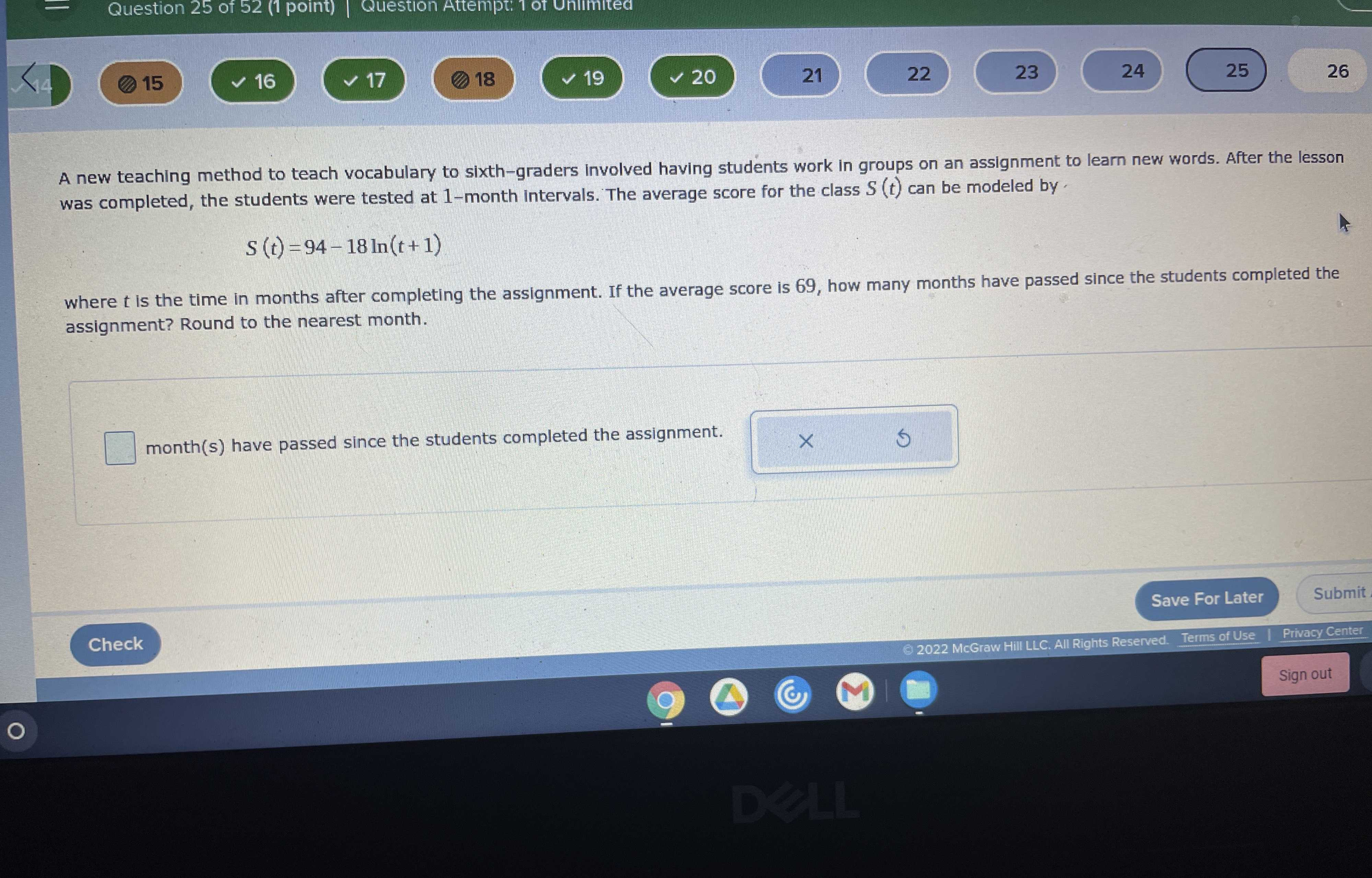### ¿Todavía tienes preguntas de matemáticas?

Pregunte a nuestros tutores expertos
Algebra
PreguntaA new teaching method to teach vocabulary to sixth-graders involved having students work in groups on an assignment to learn new words. After the lesson was completed, the students were tested at $$1$$ -month intervals. The average score for the class $$S ( t )$$ can be modeled by.

$$S ( t ) = 94 - 18 \ln ( t + 1 )$$, where $$t$$ is the time in months after completing the assignment. If the average score is $$69$$ , how many months have passed since the students completed the assignment? Round to the nearest month.

$$\square$$ month(s) have passed since the students completed the assignment.

$$S(t)= 69,t= e^{\frac{94- S(t)}{18} }- 1\approx3$$